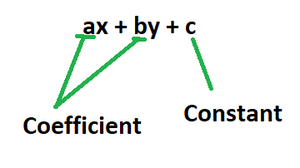GeeksforGeeks App
Open AppBrowser
Continue

# What is the coefficient of y in 3xy?

Algebra is a discipline of mathematics concerned with the study of various symbols that represent quantities that do not have a fixed value or amount associated with them but instead vary or change over time in relation to some other factor. In the study of algebra, such symbols are referred to as variables, and the numbers associated with them are referred to as coefficients. They can be represented in a variety of ways, including forms and even English alphabets. In other words, algebra studies the representation of numbers using letters or symbols rather than the representation of their actual values.

#### Algebraic Expression

In mathematics, an algebraic expression is a statement that is constructed utilizing variables and constants, as well as numerous arithmetic operations such as addition, subtraction, multiplication, division, exponential operations, root extraction such as square root, cube root, fourth root, and so on.

Examples:

• a + x
• ax + b
• ax2 + bx + y + xz + yc

### The coefficient in Algebraic Expression

A coefficient is known as a multiplicative factor in any polynomial, series, or expression; mostly, it is a number, however, it can be any expression too. This implies that in any given algebraic expression, whatever term accompanies the given variable is called the coefficient of that term.### What is the coefficient of y in 3xy?

Solution:

Clearly, 3xy is an algebraic expression in variables x and y. A coefficient is known as a multiplicative factor in any polynomial, series, or expression; mostly, it is a number, however, it can be any expression too

In this case, the multiplicative factor associated with the variable y is 3x.

Hence the coefficient of y in 3xy is 3x.

### Similar Problems

Question 1: What is the coefficient of y in 3x2y?

Solution:

Clearly, 3x2y is an algebraic expression in variables x and y. A coefficient is known as a multiplicative factor in any polynomial, series, or expression; mostly, it is a number, however, it can be any expression too

In this case, the multiplicative factor associated with the variable y is 3x2.

Hence the coefficient of y in 3x2y is 3x2.

Question 2: What is the coefficient of x in -3xy5?

Solution:

Clearly, -3xy5 is an algebraic expression in variables x and y. A coefficient is known as a multiplicative factor in any polynomial, series, or expression; mostly, it is a number, however, it can be any expression too

In this case, the multiplicative factor associated with the variable x is -3y5.

Hence the coefficient of x in -3xy5 is -3y5.

Question 3: What is the coefficient of x2 in 6 – x2?

Solution:

Clearly, 6 – x2 is an algebraic expression in variable x. A coefficient is known as a multiplicative factor in any polynomial, series, or expression; mostly, it is a number, however, it can be any expression too

In this case, the multiplicative factor associated with the variable x2 is -1.

Hence the coefficient of x2 in 6 – x2 is -1.

Question 4: What is the coefficient of x2 in?

Solution:

Clearly,is an algebraic expression in variable x. A coefficient is known as a multiplicative factor in any polynomial, series, or expression; mostly, it is a number, however, it can be any expression too

In this case, the multiplicative factor associated with the variable x2 is.

Hence the coefficient of x2 inis.

Question 5: What is the coefficient of x in -6x?

Solution:

Clearly, -6x is an algebraic expression in variable x. A coefficient is a multiplicative factor in a polynomial, a series, or any expression in mathematics; it is usually a number, although it can be any expression.

In this case, the multiplicative factor associated with the variable x is -6.

Hence the coefficient of x in -6x is -6.

My Personal Notes arrow_drop_up
Related Tutorials